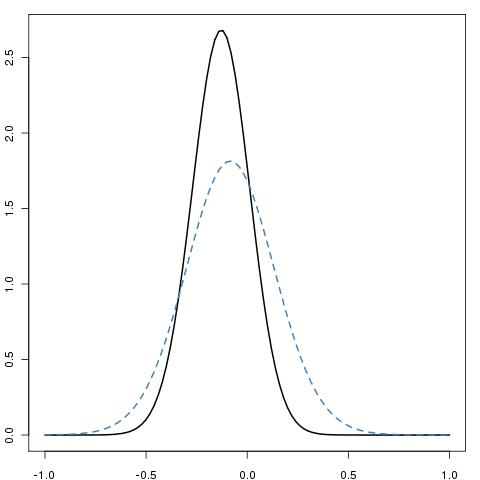# More typos in Chapter 5

December 29, 2010
By

Want to share your content on R-bloggers? click here if you have a blog, or here if you don't.Following Ashley’s latest comments on Chapter 5 of Introducing Monte Carlo Methods with R, I realised Example 5.5 was totally off-the-mark! Not only the representation of the likelihood should have used prod instead of mean, not only the constant should call the val argument of integrate, not only integrate  uses lower and upper rather than from and to, but also the approximation is missing a scale factor of 10, squared root of the sample size… The corrected R code is thus

```> cau=rcauchy(10^2)
> mcau=median(cau)
> rcau=diff(quantile(cau,c(.25,.75)))/sqrt(10^2)
> f=function(x){
+   z=dcauchy(outer(x,cau,FUN="-"))
+   apply(z,1,prod)}
> fcst=integrate(f,lower=-20,upper=20)\$val
> ft=function(x){f(x)/fcst}
> g=function(x){dt((x-mcau)/rcau,df=49)/rcau}
> curve(ft,from=-1,to=1,xlab="",ylab="",lwd=2)

and the corrected Figure 5.5 is therefore as follows. Note that the fit by the t distribution is not as perfect as before. A normal approximation would do better.This mistake is most embarrassing and I cannot fathom how I came with this unoperating program! (The more embarrassing as Cauchy‘s house is about 1k away from mine…) I am thus quite grateful to Ashley for her detailed study of this example.

R-bloggers.com offers daily e-mail updates about R news and tutorials about learning R and many other topics. Click here if you're looking to post or find an R/data-science job.
Want to share your content on R-bloggers? click here if you have a blog, or here if you don't.

Tags: , , , , , , , ,